# Two rulers

We have two rulers. Scale interval on first rulers are a spaced 1 cm and on second spaced 15 mm. Rulers are attached to each other so that they match initial divider commas. What next dividers commas coincide? Find at least three cases.

a =  30 mm
b =  60 mm
c =  90 mm

### Step-by-step explanation: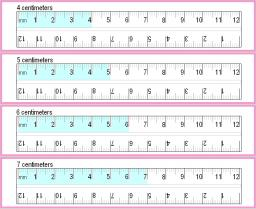Did you find an error or inaccuracy? Feel free to write us. Thank you!Tips to related online calculators
Do you want to calculate least common multiple two or more numbers?
Do you have a linear equation or system of equations and looking for its solution? Or do you have a quadratic equation?
Do you want to convert length units?

## Related math problems and questions:

• Lcm of three numbersWhat is the Lcm of 120 15 and 5
• A ropeA rope can be cut into equal length with no rope left over. The lengths can be 15cm,18cm or 25cm. What is the shortest possible length of the rope?
• PexesoDrawing paper has dimensions of 60cm and 840mm. Pupils have to divide it into squares so that they can make a pexeso. What dimension must squares have if their side is to be larger than 3cm and less than 10cm?
• LCMWhat is the least common multiple of 5, 50, 14?
• FlowerbedsWhat is the smallest length of flowerbeds we have to prepare to plant seedlings at grid 20,30,25,40 cm?
• Steps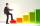Peter makes steps long 70 cm, John 45 cm long. After how many meters their footsteps meet?
• Matches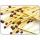George poured out of the box matches and composing them triangles and no match was left. Then he tries squares, hexagons and octagons and no match was left. How many matches must be at least in the box?
• Trams 2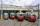Square passes two lines of tram. One running every nine minutes, a second interval of 15 minutes. Exactly at 12 o'clock arrived two tram lines in the square. How soon should a similar situation arise again?
• CubesCarol with cut bar 12 cm x 12 cm x 135 cm to the cubes. Find the sum of all the surfaces of the resulting cubes.
• Lcm simpleFind least common multiple of this two numbers: 140 175.
• Bus linesAt 5.00 in the morning, four buses left the terminal at the same time. The first bus has an interval of 15 minutes, the second 20 minutes, the third 25 minutes, and the fourth 45 minutes. How many minutes will all four buses leave the terminal at the same
• Three linesAt 6 am, three bus lines are departing from the station. The first line has an interval of 24 minutes. The second line has an interval of 15 minutes. The third line runs at regular intervals of more than 1 minute. The third line runs at the same time as t
• LCM of two numberFind the smallest multiple of 63 and 147
• Counting numberWhat is the smallest counting number divisible by 2,5,7,8, and 15?
• Tram stop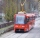The blue tram stops every 12 minutes, the red one 8 minutes. At 8 o'clock they left the stop together. How many times do they meet at a stop before 11 am?1st blade 2,5 m, 2nd blade. .1.75 m. How many same long pieces of this two blades can be do the biggest? How long is one piece?The classroom is 9 meters long. The width of the classroom is smaller and can be passed in equally long steps of 55 CM or 70 CM. Determine the width of the classroom.